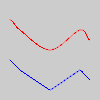# Py5Shape.curve_tightness()#

Modifies the quality of a Py5Shape object’s forms created with Py5Shape.curve_vertex().

## Examples#def setup():
py5.size(100, 100, py5.P2D)
py5.shape(draw_curves(30, 0, "#FF0000"))
py5.shape(draw_curves(70, 0.9, "#0000FF"))

def draw_curves(y, tightness, color):
s = py5.create_shape()
s.begin_shape()
s.no_fill()
s.stroke(color)
s.curve_tightness(tightness)
s.curve_vertex(0, y)
s.curve_vertex(10, y-10)
s.curve_vertex(20, y)
s.curve_vertex(50, y+20)
s.curve_vertex(80, y)
s.curve_vertex(90, y+10)
s.curve_vertex(100, y)
s.end_shape()
return s


## Description#

Modifies the quality of a Py5Shape object’s forms created with Py5Shape.curve_vertex(). The parameter tightness determines how the curve fits to the vertex points. The value 0.0 is the default value for tightness (this value defines the curves to be Catmull-Rom splines) and the value 1.0 connects all the points with straight lines. Values within the range -5.0 and 5.0 will deform the curves but will leave them recognizable and as values increase in magnitude, they will continue to deform.

Drawing 2D curves requires using the P2D renderer and drawing 3D curves requires using the P3D renderer. When drawing directly with Py5Shape objects, curves do not work at all using the default renderer.

This method can only be used within a Py5Shape.begin_shape() and Py5Shape.end_shape() pair.

Underlying Processing method: PShape.curveTightness

## Signatures#

curve_tightness(
tightness: float,  # amount of deformation from the original vertices
/,
) -> None


Updated on March 06, 2023 02:49:26am UTC